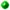# Each Way BettingAn each way bet is actually two bets. One bet is that the horse will win the race and is settled in the same way as a win only bet using the quoted starting price (SP), as follows:

Return = Stake × (SP + 1)

The second bet is that the horse will be 'placed' and is settled using a fraction of the SP, as follows:

Return = Stake × ( (SP × F) + 1)

Where F is the relevant fraction determined by the size and type of race (see below). F will be either 1, 1/4 or 1/5.

The total return from the each way bet is the return of the win part and the place part added together. For the champion Tipster competition the stake is 1 point (i.e. two points are staked on each selection - one for win and one for place). Therefore, the points awarded for a horse that wins is :

Points = (SP + 1) + ( (SP × F) + 1)

If a horse is just placed, the points awarded are:

Points = (SP × F) + 1

Whether the horse is placed and what fraction of the SP is used depends upon the size and type of race. Specifically, the number of horses in the race and whether the race is a handicap.

A horse is placed if any of the following four conditions is true:

1. The horse wins the race.
2. The horse finishes second and the race has five or more runners.
3. The horse finishes third and the race has more than eight runners.
4. The horse finishes fourth and the race has more than sixteen runners AND the race is a handicap.
The fraction of the SP used to settle the bet (F) also depends upon the size and type of race as follows:Fraction is 1 (i.e. full SP) if the race has 4 or less runners.Fraction is 1/4 if the race has 5 to 7 (inclusive) runners.Fraction is 1/5 if the race has 8 to 11 (inclusive) runners.Fraction is 1/5 if the race has 12 or more runners AND is not a handicap.Fraction is 1/4 if the race has 12 or more runners AND is a handicap.
This table summarises the place rules:

No Of Runners Race Type Places Fraction
4 or less All 1 1
5-7 All 1 & 2 1/4
8 or more Non-Handicap 1, 2 & 3 1/5
8-11 Handicap 1, 2 & 3 1/5
12-15 Handicap 1, 2 & 3 1/4
16 or more Handicap 1, 2, 3 & 4 1/4

## EXAMPLES

The 1:40 at Sandown was a handicap and had 5 runners. Giocoso came second with a starting price of 15/8. Points awarded were:

(15/8 × 1/4) + 1 = 1.47 points

The 2:05 at Haydock was not an handicap and had 8 runners. Every Note Counts won the race at 11/2. Points were:

(11/2 + 1) + ( (11/2 × 1/5) + 1) = 6.5 + 2.1 = 8.6 points

## HAVE YOU MADE A KILLING?

It may be interesting to work out whether you would have made any money if you had bet on your selections in a real betting shop rather than just playing the Champion Tipster competition. To check this, multiply the number of selections you have made by two and take this away from the number of points you have. Multiply the result by the amount of money you would have staked on each race. If the result is positive, this is the amount you would have won. If the result is negative, this is what you would have lost! As an example, Tony made 13 selections in the 2004 Champion Tipster competition and scored a total of 41.1 points. If he had put a Ł5 each way bet on each selection, his return would have been:

5 × (41.1 – (13 × 2)) = 75.5

That is, he would have won Ł75.50. Not bad for a summers work!